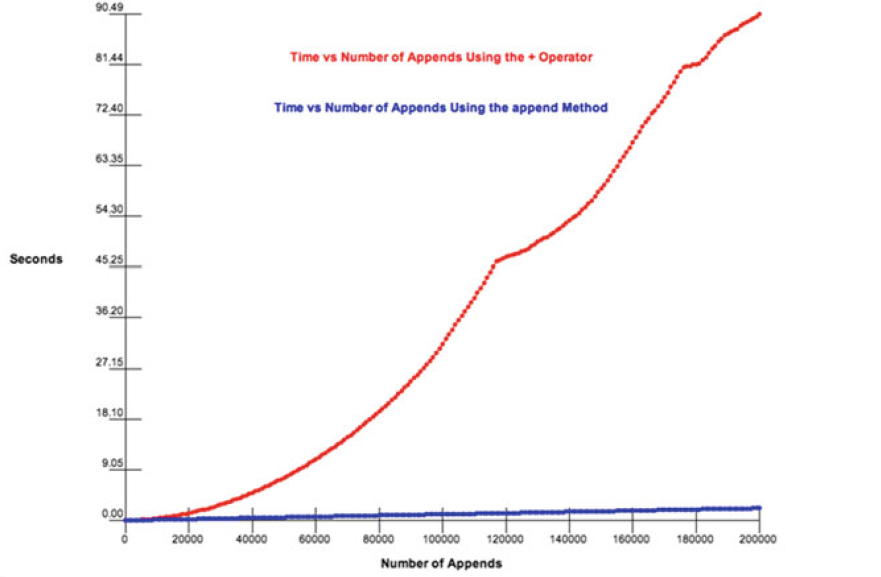# Difference Between ‘+’ and ‘append’ in Python

Using ‘+’ operator to add an element in the list in Python: The use of the ‘+’ operator causes Python to access each element of that first list. When ‘+’ is used a new list is created with space for one more element. Then all the elements from the old list must be copied to the new list and the new element is added at the end of this list.

Example:

 `sample_list ``=``[] ` `n ``=` `10` ` `  `for` `i ``in` `range``(n): ` `     `  `    ``# i refers to new element ` `    ``sample_list ``=` `sample_list``+``[i] ` ` `  `print``(sample_list) `

Output:

`[0, 1, 2, 3, 4, 5, 6, 7, 8, 9]`
• The ‘+’ operator refers to the accessor method and does not modify the original list.
• In this, sample_list doesn’t change itself. This type of addition of element in sample_list creates a new list from the elements in the two lists.
• The assignment of sample_list to this new list updates PythonList object so it now refers to the new list.

#### Complexity to add n elements

Have you wondered, how it works as the size of the Python List grows? Let us see with the explanation.

For every ith iteration, there will have to be i elements copied from the original list to form a new list. Considering the time taken to access an element from a list to be constant. So, the complexity or amount of time it takes to append n elements to the Python List i.e. sample_list we would have to add up all the list accesses and multiply by the amount of time it takes to access a list element plus the time it takes to store a list element. To count the total number of access and store operations we must start with the number of access and store operations for copying the list the first time an element is appended. That’s one element copied. The second append requires two copy operations. The third append requires three copy operations. So, we have the following number of list elements being copied.Therefore, time complexity=O()

Using .append() method i.e. an efficient approach: The .append() method on lists changes the code to use a mutator method to alter the list by appending just one more element.

Example:

 `sample_list ``=``[] ` `n ``=` `10` ` `  `for` `i ``in` `range``(n): ` `    ``# i refers to new element ` `    ``sample_list.append(i)  ` ` `  `print``(sample_list) `

Output:

```[0, 1, 2, 3, 4, 5, 6, 7, 8, 9]
```

It turns out that adding one more element to an already existing list is very efficient in Python. In fact, adding a new item to a list is an O(1) operation.

So overall complexity to append n elements is

`1+.....(n-2) times...+1=O(n)`

Note: Proof that `.append()` method has O(1) complexity to add new element is given by the accounting method to find the amortized complexity of append.

### Graphical comparison between ‘+’ and ‘append’My Personal Notes arrow_drop_upCheck out this Author's contributed articles.

If you like GeeksforGeeks and would like to contribute, you can also write an article using contribute.geeksforgeeks.org or mail your article to contribute@geeksforgeeks.org. See your article appearing on the GeeksforGeeks main page and help other Geeks.

Please Improve this article if you find anything incorrect by clicking on the "Improve Article" button below.

Article Tags :
Practice Tags :

2

Please write to us at contribute@geeksforgeeks.org to report any issue with the above content.